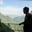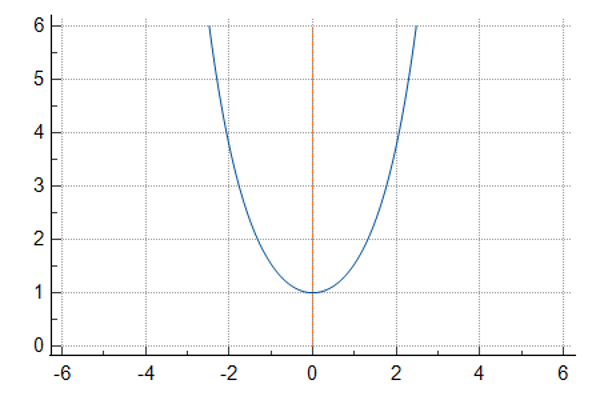Related Tags

sql
hyperbolic cosine
cosh
communitycreator

# What is COSH( ) in SQL?AKASH BAJWA

This COSH() function returns the hyperbolic cosine of a number. It accepts an angle β in radian measure, but it should be a number.

Figure 1 shows the mathematical representation of the hyperbolic cosine method.

Figure 2 represents the graphical behavior of COSH() function between -3 and +3 intervals.

Figure 1 : Mathematical Representation of COSH(θ)Figure#2 Graphical Illustration of COSH(β)

### Syntax

COSH(β) -- β is an angle measured in radians.


To convert degrees to radians, use this formula:

Radian angle = $\frac{x\pi}{180}$, where $\pi$ = 3.14 and $x$ is the degree angle.

### Parameter

This COSH() method requires a floating-point number as an argument.

Usually, this double-precision floating-point number takes 64-bits of computer memory.

### Return value

The COSH() function returns the hyperbolic cosine of the value that is sent as an argument.

• Passing NULL as a parameter to COSH() function, the function returns NULL.
• Passing 0 as a parameter to COSH() function, the function returns 1.0.

### Code

-- Example showing how to use COSH(β)

/*Positive number*/
SELECT COSH(4); -- return 27.308232836016

/*Negative number*/
select COSH(-1); -- return 1.543080634815

/*Fractional number*/
select COSH(0.8);  -- return 1.337434946305

RELATED TAGS

sql
hyperbolic cosine
cosh
communitycreator

CONTRIBUTORAKASH BAJWA
RELATED COURSES

View all Courses

Keep Exploring

Learn in-demand tech skills in half the time